Document Type : Original Research Article

Authors

1 MCS, National University of Sciences and Technology, Islamabad, Pakistan

2 Department of Mathematics and Statistics, The University of Lahore, Lahore, 54000, Pakistan

3 Department of Applied Mathematics, Iran University of Science and Technology (IUST), Narmak, Tehran 16844, Iran

4 Faculty of Education, Van Yuzuncu Yıl University, Zeve Campus, Tuşba, 65080, Van, Turkey

Abstract

Chemical graph theory is one of the dominant branches in graph theory. In this paper, we compute the atom bond connectivity, geometric arithmetic, first K-Banhatti, second K-Banhatti, first K-hyper Banhatti, second K-hyper Banhatti, modified first K-Banhatti, modified second K-Banhatti and harmonic K-Banhatti index via M-polynomial of zig-zag Benzenoid system. We also elaborate the result with graphical representation.

Graphical AbstractKeywords

Main Subjects

###### ##### Full Text

Introduction

Graph theory is one of the most popular and versatile fields of mathematics and has innumerable applications in diverse fields of science like physics, chemistry, operational research, biology and computer science . In chemical graphs, topological indices have great importance. Chemical graph theory is the sub-study of graph theory which reviews and examines the chemical structures of the chemical compounds. A chemical graph basically models the chemical system used to show the interactions like its components, such as atoms, bonds and molecules. It is also called a reaction graph.

In mathematical chemistry, a mathematical tool such as polynomials and numbers predict properties of compounds without utilizing quantum mechanics. These compounds contain information hidden in the molecule.  The most common known invariants of such types are called degree-based topological index.

The first topological index was introduced by wiener . Topological indices are arithmetic numbers about a graph of the chemical molecules. Every molecule is normally shown by a set of topological indices. Topological variants are derived from hydrogen-suppressed molecular graphs. In theoretical chemistry many topological indices are used to inspect the properties of molecules which are divided into three classes as follows: Distance-dependent, degree-dependent and counted related topological indices. The topological indices also are computed via M-polynomial. Several previous works can be found on this [3-6, 10-20].  Let us define some topological indices.

Atom bond connectivity index

The atom bond connectivity index (ABC) is a molecular structure pattern that has recently provided a principal application in rationalizing the stability of linear and separate alkanes like as strain energy of cycloalkanes.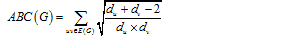Geometric arithmetic index

In 2009, Damir Vukičević and Boris Furtula used a new index known as geometric arithmetic index .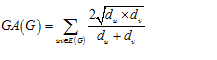K-Banhatti and K-hyper Banhatti index

In 2016, V R Kulli proposed some indices [21, 22], named as first K-Banhatti index, second K-Banhatti index, first K-hyper Banhatti index and second K-hyper Banhatti index, defined as First K-Banhatti index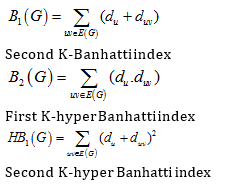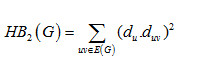Modified K-Banhatti and Harmonic K-Banhatti index

In 2017, V R Kulli introduced some more indices , named as modified first K-Banhatti index, modified second K-Banhatti index and Harmonic K-Banhatti index, and defined as: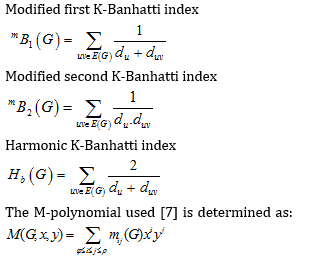Where φ=max{dv: vV(G)}, ρ=min{dv: vV(G)} and mjk(G) is the total number of edges vuE(G) where {dv,du}={j,k}. In last few year many topological indices with the help of M-polynomial have been computed [1,2,4,10-20].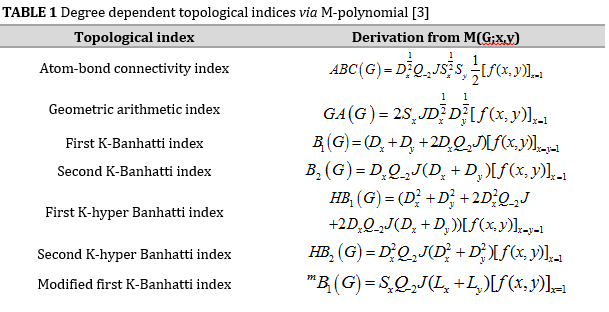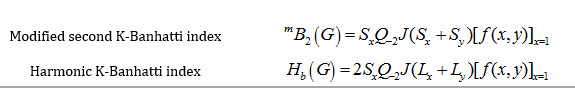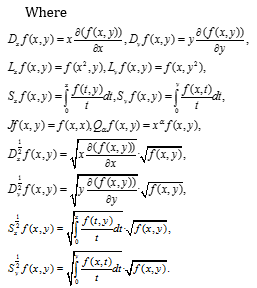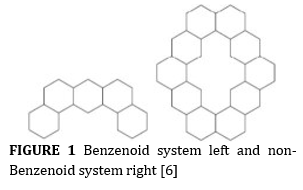Zig-zag Benzenoid System

Benzenoid hydrocarbon plays a vital role in our environment and in the food and chemical industry. Benzenoid molecular graphs are systems with deleted hydrogens. Let n represents the number of rows in a graph of zig-zag Benzenoid system Zn with every row contains two hexagons. The first row contains two hexagons with twelve edges and one edge is common, so we obtain eleven edges in the first row. If we add first and second row, we obtain 24 total edges with three edges that are common, after that we obtain total 21 edges in both rows. Continuing in the same way, we obtain 10n+1 edges and 8n+2 vertices.

Consider the zig-zag Benzenoid system Zn.  The Benzenoid system and non-Benzenoid system are shown in Figure 1. The M-polynomial of Zn which have been compute in  is represented as

M (Zn;m,n)=2(n+2)s2t2+4ns2t3+(4n-3)s3t3.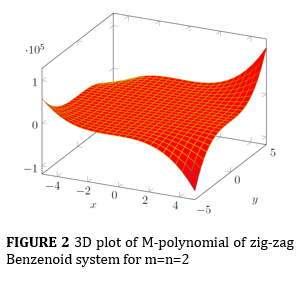Topological indices of Zig-zag Benzenoid system

Theorem 1. Let Zn be the zig-zag Benzenoid system, then its M-polynomials is

M(Zn; m; n)=(2n+4)s2t2+4ns2t3+(4n-3)s3t

Then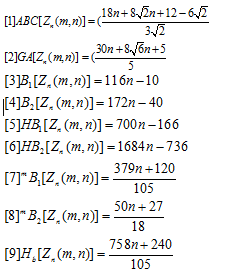Proof. Let

M(Zn;m;n)=(2n+4)s2t2+4ns2t3+(4n-3)s3t3  and by using results from Table 1, we will have: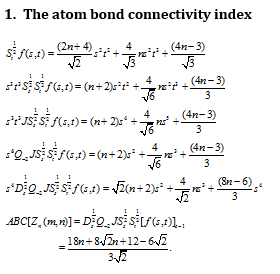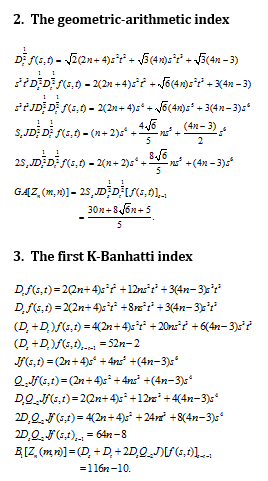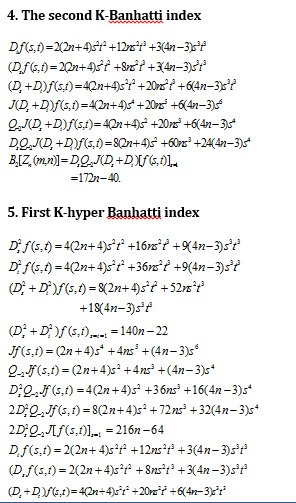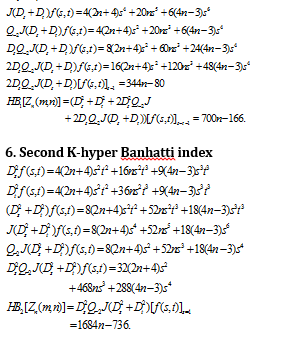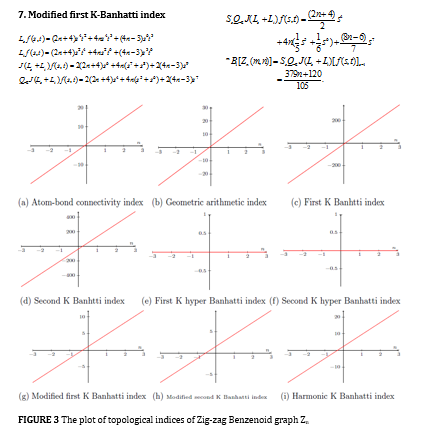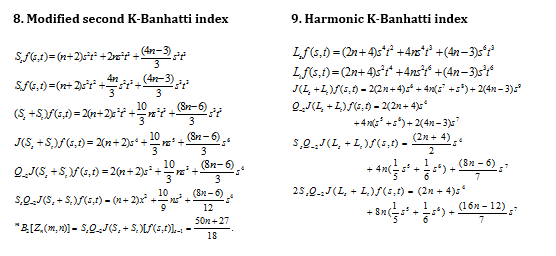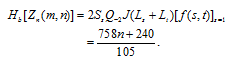Conclusion

In this paper, we computed the degree-based topological indices via M-polynomial of Benzenoid system. This polynomial was improved to calculate the topological indices values of the molecular structure. We plotted graphs of M-polynomial against the number of hexagon n in every diagram. These indices play a principal role in finding the properties of the chemical compounds.

Graphical analyses

The 3-dimensional and 2-dimensional plots of M-polynomial of Zig-zag Benzenoid graph Zn are shown in figures 2 and 3, respectively.

Acknowledgments

The authors would like to thank the reviewers for their helpful suggestions and comments.

Orcid:

Farkhanda Afzal: https://orcid.org/0000-0001-5396-7598

Deeba Afzal: https://orcid.org/0000-0001-5268-7260

https://orcid.org/0000-0003-2969-4280

Murat Cancan: https://orcid.org/0000-0002-8606-2274

 How to cite this article: Farkhanda Afzal, Muhammad Zaman, Faryal Chaudhry, Deeba Afzal*, Mohammad Reza Farahani, Murat Cancan. Computational analysis of new degree-based descriptors of Zig-Zag Benzenoid system. Eurasian Chemical Communications, 2021, 3(6), 418-424. Link: http://www.echemcom.com/article_130834.html

###### ##### References
 D. Afzal, S. Hussain, M. Aldemir, M. Farahani, F. Afzal, Eurasian Chem. Commun., 2020, 2, 1117-1125. [crossref], [Google Scholar], [Publisher]
 F. Afzal, S. Hussain, D. Afzal, S. Hameed. Open Chem., 2020, 18, 1362-1369. [crossref], [Google Scholar], [Publisher]
 F. Afzal, S. Hussain, D. Afzal, S. Razaq. J. Inf. Opt. Sci., 2020, 41, 1061-1076. [crossref], [Google Scholar], [Publisher]
 A. Ali, W. Nazeer, M. Munir, S.M. Kang, Open Chem., 2018, 16, 73-78. [crossref], [Google Scholar], [Publisher]
 M. Cancan, D. Afzal, S. Hussain, A. Maqbool, F. Afzal, J. D. Math. Sci. Cryp., 2020, 23, 1157-1171. [crossref], [Google Scholar], [Publisher]
 M. Cancan, S. Ediz, H. Mutee-Ur-Rehman, D. Afzal, J. Inf. Opt. Sci., 2020, 41, 1117-1131. [crossref], [Google Scholar], [Publisher]
 E. Deutsch, S. Klavžar, Iran. J. Math. Chem., 2015, 6, 93-102. [crossref], [Google Scholar], [Publisher]
 S.M. Kang, W. Nazeer, W. Gao, D. Afzal, S.N. Gillani, Open Chem., 2018, 16, 201-213. [crossref], [Google Scholar], [Publisher]
 A.J.M. Khalaf, S. Hussain, D. Afzal, F. Afzal, A. Maqbool, J. D. Math. Sci. Cryp., 2020, 23, 1217-1237. [crossref], [Google Scholar], [Publisher]
 W. Gao, M.R. Farahani, J. Nanotech., 2016, 2016, 1-6. [crossref], [Google Scholar], [Publisher]
 H. Yang, A.Q. Baig, W. Khalid, M.R. Farahani, X. Zhang, J. Chem., 2019, 2019, Article ID 7297253, 1-9. [crossref], [Google Scholar], [Publisher]
 M.R. Farahani, World Appl. Sci. J., 2012, 20, 1248-1251. [crossref], [Google Scholar], [Publisher]
 M. Imran, S.A. Bokhary, S. Manzoor, M.K. Siddiqui, Eurasian Chem. Communm., 2020, 2, 680-687. [crossref], [Google Scholar], [Publisher]
 Z. Ahmad, M. Naseem, M.K. Jamil, Sh. Wang, M.F. Nadeem, Eurasian Chem. Commun., 2020, 2, 712-721. [crossref], [Google Scholar], [Publisher]
 M.R. Farahani, Acta Chim. Slov., 2012, 59, 965-968. [Pdf], [Google Scholar], [Publisher]
 D.Y. Shin, S. Hussain, F. Afzal, C. Park, D. Afzal, M.R. Farahani, Front. Chem., 2021, 8, Article 613873, 1-6. [crossref], [Google Scholar], [Publisher]
 M. Cancan, S. Ediz, M.R. Farahani, Eurasian Chem. Commun., 2020, 2, 641-645. [crossref], [Google Scholar], [Publisher]      A.Q. Baig, M. Naeem, W. Gao, J.B. Liu, Eurasian Chem. Commun., 2020, 2, 634-640. [crossref], [Google Scholar], [Publisher]
 F. Afzal, M.A. Razaq, D. Afzal, S. Hameed, Eurasian Chem. Commun., 2020, 2, 652-662. [crossref], [Google Scholar], [Publisher]
 M. Alaeiyan, C. Natarajan, G. Sathiamoorthy, M.R. Farahani, Eurasian Chem. Commun., 2020, 2, 646-651. [crossref], [Google Scholar], [Publisher]
 V. Kulli, J. Comput Math. Sci., 2016, 7, 213-218. [Pdf], [Google Scholar], [Publisher]
 V. Kulli, Int. Res. J. Pure Algebra, 2016, 6, 300-304.
 V. Kulli, Int. J.Fuzzy Math. Arch., 2017, 12, 29-37. [crossref], [Google Scholar], [Publisher]
 S.G. Shirinivas, S. Vetrivel, N.M. Elango, Int. J. Eng. Sci. Tech., 2010, 2, 4610-4621. [Pdf], [Google Scholar], [Publisher]
 D. Vukičević, B. Furtula, J. Math. Chem., 2009,46,1369–1376. [crossref], [Google Scholar]
 H. Wiener, J. Am. Chem. Soc., 1947,69, 17-20. [crossref], [Google Scholar], [Publisher